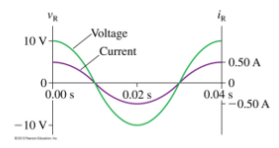# Problem: a) What is the emf frequency f? b) What is the value of the resistance R? c) Draw the resistor's voltage and current phasors at t = 15 ms

###### FREE Expert Solution

Frequency:

$\overline{){\mathbf{f}}{\mathbf{=}}\frac{\mathbf{1}}{\mathbf{T}}}$

From Ohm's law:

$\overline{){{\mathbf{i}}}_{{\mathbf{0}}}{\mathbf{R}}{\mathbf{=}}{{\mathbit{\epsilon }}}_{{\mathbf{0}}}}$

a)

From the figure, the period, T = 0.04s

The period is the time taken for full oscillation.

79% (312 ratings)###### Problem Detailsa) What is the emf frequency f?

b) What is the value of the resistance R?

c) Draw the resistor's voltage and current phasors at t = 15 ms

Frequently Asked Questions

What scientific concept do you need to know in order to solve this problem?

Our tutors have indicated that to solve this problem you will need to apply the Phasors for Resistors concept. You can view video lessons to learn Phasors for Resistors. Or if you need more Phasors for Resistors practice, you can also practice Phasors for Resistors practice problems.

What professor is this problem relevant for?

Based on our data, we think this problem is relevant for Professor Phipps' class at CSUEASTBAY.